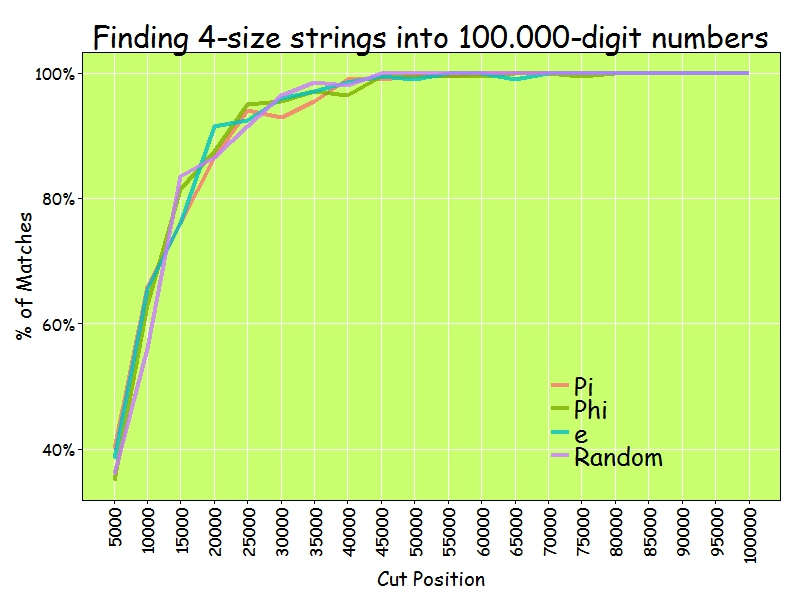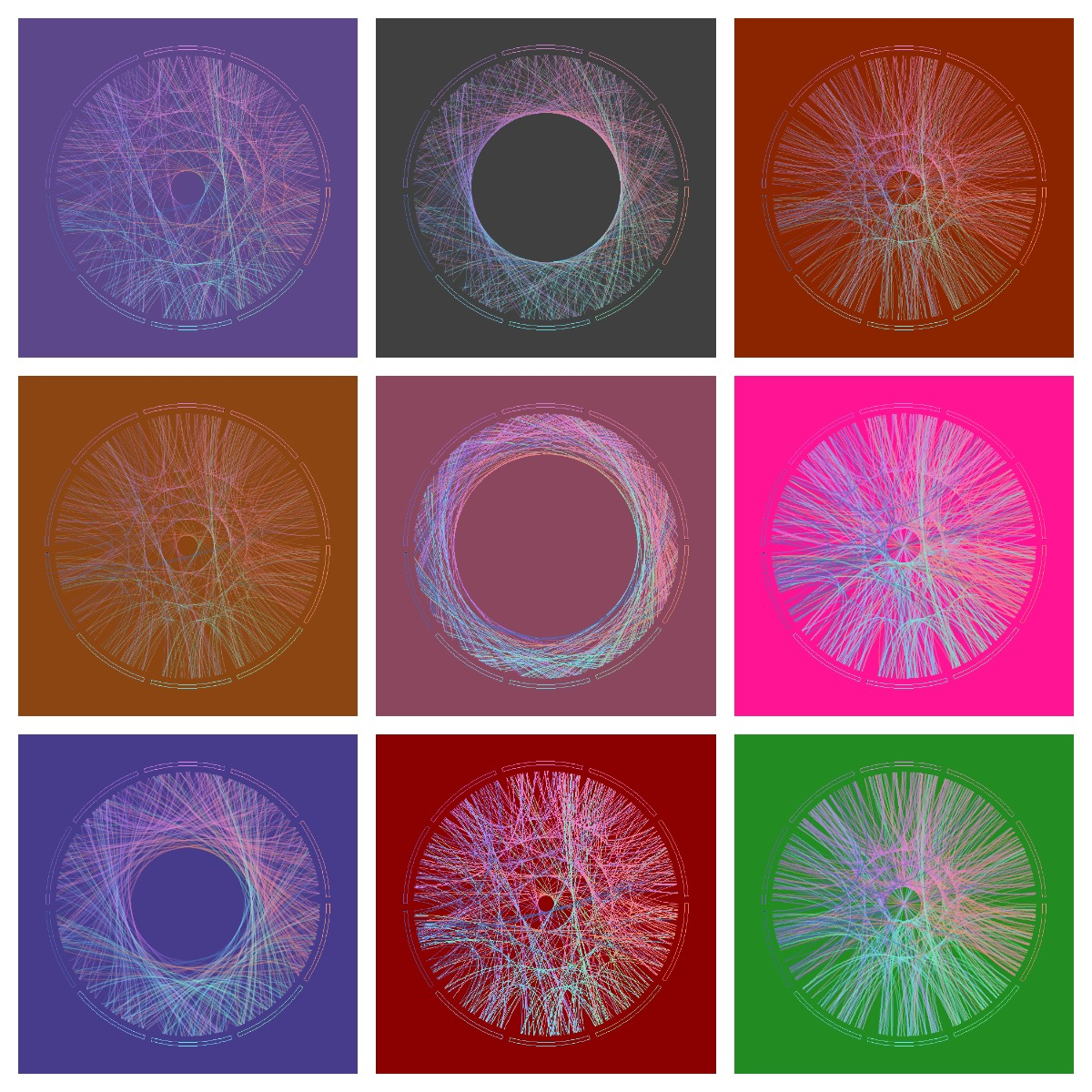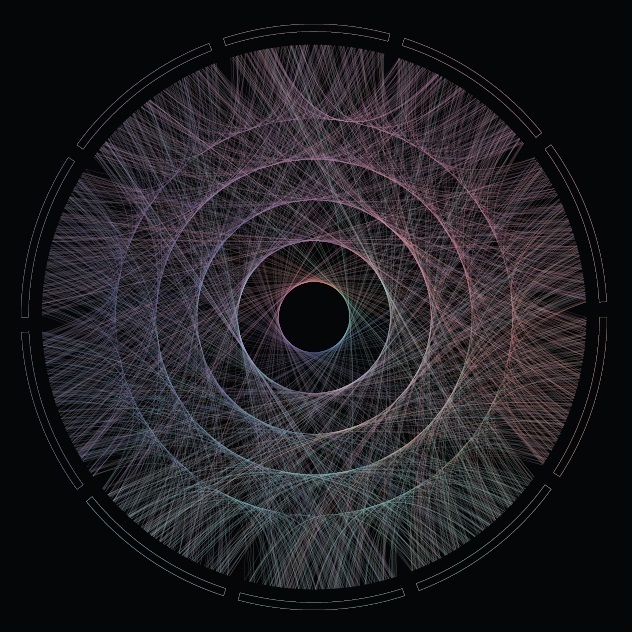# Going Bananas #2: A Needle In A Haystack

Now I’m gonna tell my momma that I’m a traveller, I’m gonna follow the sun (The Sun, Parov Stelar)

Inspired by this book I read recently, I decided to do this experiment. The idea is comparing how easy is to find sequences of numbers inside Pi, e, Golden Ratio (Phi) and a randomly generated number. For example, since Pi is 3.1415926535897932384… the 4-size sequence 5358 can be easily found at the begining as well as the 5-size sequence 79323. I considered interesting comparing Pi with a random generated number. What I though before doing the experiment is that it would be easier finding sequences inside the andom one. Why? Because despite of being irrational and transcendental I thought there should be some kind of residual pattern in Pi that should make more difficult to find random sequences inside it than do it inside a randomly generated number.

• I downloaded Pi, e and Phi from the Internet and extract first 100.000 digits of all of them. I generate a random 100.000 number on the fly.
• I generate a representative sample of 4-size sequences
• I look for each of these sequences inside first 5.000 digits of Pi, e, Phi and the randomly generated one. I repeat searching for first 10.000, first 15.000 and so on until I search into the whole 100.000 -size number
• I store how many sequences I find for each searching
• I repeat this for 5 and 6-size sequences.

At first sight, is equally easy (or difficult), to find random sequences inside all numbers: my hypothesis was wrong.

As you can see here, 100.000 digits is more than enough to find 4-size sequences. In fact, from 45.000 digits I reach 100% of successful matches:I only find 60% of 5-size sequences inside 100.000 digits of numbers:And only 10% of 6-size sequences:Why these four numbers are so equal in order to find random sequences inside them? I don’t know. What I know is that if you want to find your telephone number inside Pi, you will probably need an enormous number of digits.

```library(rvest)
library(stringr)
library(reshape2)
library(ggplot2)
library(extrafont);windowsFonts(Comic=windowsFont("Comic Sans MS"))
library(dplyr)
library(magrittr)
library(scales)
p = html("http://www.geom.uiuc.edu/~huberty/math5337/groupe/digits.html")
e = html("http://apod.nasa.gov/htmltest/gifcity/e.2mil")
p %>%
html_text() %>%
substr(., regexpr("3.14",.), regexpr("Go to Historical",.)) %>%
gsub("[^0-9]", "", .)  %>%
substr(., 1, 100000) -> p
f %>%
html_text() %>%
substr(., regexpr("1.61",.), nchar(.)) %>%
gsub("[^0-9]", "", .) %>%
substr(., 1, 100000) -> f
e %>%
html_text() %>%
substr(., regexpr("2.71",.), nchar(.)) %>%
gsub("[^0-9]", "", .) %>%
substr(., 1, 100000) -> e
r = paste0(sample(0:9, 100000, replace = TRUE), collapse = "")
results=data.frame(Cut=numeric(0), Pi=numeric(0), Phi=numeric(0), e=numeric(0), Random=numeric(0))
bins=20
dgts=6
samp=min(10^dgts*2/100, 10000)
for (i in 1:bins) {
cut=100000/bins*i
p0=substr(p, start=0, stop=cut)
f0=substr(f, start=0, stop=cut)
e0=substr(e, start=0, stop=cut)
r0=substr(r, start=0, stop=cut)
sample(0:(10^dgts-1), samp, replace = FALSE) %>% str_pad(dgts, pad = "0") -> comb
comb %>% sapply(function(x) grepl(x, p0)) %>% sum() -> p1
comb %>% sapply(function(x) grepl(x, f0)) %>% sum() -> f1
comb %>% sapply(function(x) grepl(x, e0)) %>% sum() -> e1
comb %>% sapply(function(x) grepl(x, r0)) %>% sum() -> r1
results=rbind(results, data.frame(Cut=cut, Pi=p1, Phi=f1, e=e1, Random=r1))
}
results=melt(results, id.vars=c("Cut") , variable.name="number", value.name="matches")
opts=theme(
panel.background = element_rect(fill="darkolivegreen1"),
panel.border = element_rect(colour="black", fill=NA),
axis.line = element_line(size = 0.5, colour = "black"),
axis.ticks = element_line(colour="black"),
panel.grid.major = element_line(colour="white", linetype = 1),
panel.grid.minor = element_blank(),
axis.text.y = element_text(colour="black"),
axis.text.x = element_text(colour="black"),
text = element_text(size=20, family="Comic"),
legend.text = element_text(size=25),
legend.key = element_blank(),
legend.position = c(.75,.2),
legend.background = element_blank(),
plot.title = element_text(size = 30))
ggplot(results, aes(x = Cut, y = matches/samp, color = number))+
geom_line(size=1.5, alpha=.8)+
scale_color_discrete(name = "")+
scale_x_continuous(breaks=seq(100000/bins, 100000, by=100000/bins))+
scale_y_continuous(labels = percent)+
theme(axis.text.x = element_text(angle = 90, vjust=.5, hjust = 1))+
labs(title=paste0("Finding ",dgts, "-size strings into 100.000-digit numbers"),
x="Cut Position",
y="% of Matches")+opts
```

# Discovering Shiny

It is not an experiment if you know it is going to work (Jeff Bezos)

From time to time, I discover some of my experiments translated into Shiny Apps, like this one. Some days ago, I discovered one of these translations and I contacted the author, who was a guy from Vietnam called Vu Anh. I asked him to do a Shiny App from this experiment. Vu was enthusiastic with the idea. We defined some parameters to play with shape, number, width and alpha of lines as well as background color and I received a perfect release of the application in just a few hours. With just a handful of parameters, possible outputs are almost infinite. Following you can find some of them:I think the code is a nice example to take the first steps in Shiny. If you are not used to Markdown files, you can follow this instructions to run the code.

Vu is a talented guy, who loves maths and programming. He represents the future of our nice profession and I predict a successful future for him. Do not miss his brand new blog. I am sure you will find amazing things there.

This is the code of the app:

```---
title: "Maths, Music and Merkbar"
author: "Brother Rain"
date: "18/03/2015"
output: html_document
runtime: shiny
---

```{r}
library(circlize)
library(scales)
factors = as.factor(0:9)
lines = 2000 #Number of lines to plot in the graph
alpha = 0.4  #Alpha for color lines
colors0=c(
rgb(239,143,121, max=255),
rgb(126,240,188, max=255),
rgb(111,228,235, max=255),
rgb(127,209,249, max=255),
rgb( 74,106,181, max=255),
rgb(114,100,188, max=255),
rgb(181,116,234, max=255),
rgb(226,135,228, max=255),
rgb(239,136,192, max=255),
rgb(233,134,152, max=255)
)
# You can find the txt file here:
```

## Visualization

```{r, echo=FALSE}
fluidPage(
fluidRow(
column(width = 4,
sidebarPanel(
sliderInput("lines", "Number of lines:", min=100, max=100000, step=100, value=500),
sliderInput("alpha", "Alpha:", min=0.01, max=1, step=0.01, value=0.4),
sliderInput("lwd", "Line width", min=0, max=1, step=0.05, value=0.2),
selectInput("background", "Background:",
c("Purple" = "mediumpurple4", "Gray" = "gray25", "Orange"="orangered4",
"Violet"="palevioletred4", "Green"="forestgreen", "Pink"="deeppink"), selected="Purple"),
sliderInput("h0", "h0:", min=0, max=0.4,
step=0.0005, value=0.1375),
sliderInput("h1", "h1:", min=0, max=0.4,
step=0.0005, value=0.1125),
width=12
)
),
column(width = 8,
renderPlot({
# get data
phi=gsub("\\.","", substr(phi,1,input\$lines))
phi=gsub("\\.","", phi)
position=1/(nchar(phi)-1)

# create circos
circos.clear()
par(mar = c(1, 1, 1, 1), lwd = 0.1,
cex = 0.7, bg=alpha(input\$background, 1))
circos.par(
"track.height" = 0.025,
"gap.degree" = 3
)
circos.initialize(factors = factors, xlim = c(0, 1))
circos.trackPlotRegion(factors = factors, ylim = c(0, 1))
## create first region
for (i in 0:9) {
circos.updatePlotRegion(
sector.index = as.character(i),
bg.col = alpha(input\$background, 1),
bg.border=alpha(colors0[i+1], 1)
)
}
for (i in 1:(nchar(phi)-1)) {
m=min(as.numeric(substr(phi, i, i)), as.numeric(substr(phi, i+1, i+1)))
M=max(as.numeric(substr(phi, i, i)), as.numeric(substr(phi, i+1, i+1)))
d=min((M-m),((m+10)-M))
col=t(col2rgb(colors0[(as.numeric(substr(phi, i, i))+1)]))
for(index in 1:3){
col[index] = max(min(255, col[index]), 0)
}
if (d>0) {
substr(phi, i, i), position*(i-1),
substr(phi, i+1, i+1), position*i,
h = input\$h0 * d + input\$h1,
lwd=input\$lwd,
col=alpha(rgb(col, max=255), input\$alpha), rou = 0.92
)
}
}
}, width=600, height=600, res=192)
)
)
)

```
```

# Maths, Music and Merkbar

Control is what we already know. Control is where we have already ventured. Control is what helps us predict the future. (Merkbar)

Maths and music get along very well. Last December I received a mail from a guy called Jesper. He is one of the two members of Merkbar: a electronic music band from Denmark. As can be read in their website:

Merkbar is Jesper and David who are both interested in the psychedelic worlds and oriental spiritualism. They both studied Computer Music, where they’ve done research in sound synthesis, generative composition and the design of new digital instrument.

They asked me a front cover for their new album which will be released at the beginning of 2015. Why? Because they liked this post I did about circlizing numbers.  To do this plot I circlized the Golden Ratio number (Phi). But in this case I changed ribbons (all equal pairs of consecutive numbers gather together) by lines (every pair of consecutive numbers form a different line). As I did before, I used `circlize` package, which implements in R the features of Circos, a software to create stunning circular visualizations.

The final plot represents the first 2.000 digits of Phi:You can hear an advancement of their new album here, which is called “Phi”. Enjoy their sensitive and full-of-shades music: you will be delightfully surprised as I was.

This is the code to circlize Phi:

```library(circlize)
library(scales)
factors = as.factor(0:9)
lines = 2000 #Number of lines to plot in the graph
alpha = 0.4  #Alpha for color lines
colors0=c(rgb(239,143,121, max=255), rgb(126,240,188, max=255), rgb(111,228,235, max=255),
rgb(127,209,249, max=255), rgb( 74,106,181, max=255), rgb(114,100,188, max=255),
rgb(181,116,234, max=255), rgb(226,135,228, max=255), rgb(239,136,192, max=255),
rgb(233,134,152, max=255))
#You can find the txt file here: http://www.goldennumber.net/wp-content/uploads/2012/06/Phi-To-100000-Places.txt
phi=gsub("\\.","", substr(phi,1,lines))
phi=gsub("\\.","", phi)
position=1/(nchar(phi)-1)
#This code generates a pdf file in your working directory
pdf(file="CirclizePhi.pdf", width=25, height=25)
circos.clear()
par(mar = c(1, 1, 1, 1), lwd = 0.1, cex = 0.7, bg=alpha("black", 1))
circos.par("cell.padding"=c(0.01,0.01), "track.height" = 0.025, "gap.degree" = 3)
circos.initialize(factors = factors, xlim = c(0, 1))
circos.trackPlotRegion(factors = factors, ylim = c(0, 1))
for (i in 0:9) {circos.updatePlotRegion(sector.index = as.character(i), bg.col = alpha("black", 1), bg.border=alpha(colors0[i+1], 1))}
for (i in 1:(nchar(phi)-1)) {
m=min(as.numeric(substr(phi, i, i)), as.numeric(substr(phi, i+1, i+1)))
M=max(as.numeric(substr(phi, i, i)), as.numeric(substr(phi, i+1, i+1)))
d=min((M-m),((m+10)-M))
col=t(col2rgb(colors0[(as.numeric(substr(phi, i, i))+1)]))
if (col>255) col=255;if (col>255) col=255;if (col>255) col=255
if (col<0) col=0;if (col<0) col=0;if (col<0) col=0 if (d>0) circos.link(substr(phi, i, i), position*(i-1), substr(phi, i+1, i+1), position*i, h = 0.1375*d+0.1125, lwd=0, col=alpha(rgb(col, max=255), alpha), rou = 0.92)
}
dev.off()
```